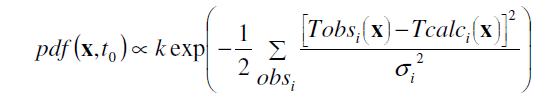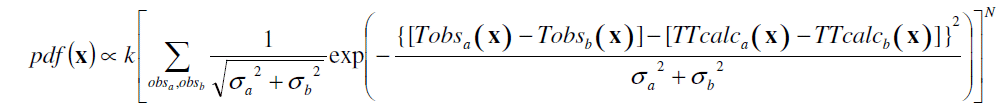## The Equal Differential Time (EDT) likelihood function

A likelihood function that is much more robust in the presence of outliers than the usual, root-mean-square, L2 norm.

NLL constructs an estimate of the location pdf within the framework of the probabilistic earthquake location methods of Tarantola and Valette (1982), Moser, van Eck and Nolet (1992) and Wittlinger et al. (1993). NLL makes available two different likelihood functions to build the pdf. The first function, LS-L2, incorporates the familiar least-squares, L2 norm (LS-L2), constructed following the formulation of Tarantola and Valette (1982). The second function, EDT, is based on a generalization by Font et al. (2004) of the Equal-Differential-Time (EDT) formulation of
Zhou(1994); all of these are extensions of the "method of hyperbolas" cited by Milne (1886). The EDT likelihood function is much more robust in the presence of outliers in the data than are the LS-L2 or other L1 and L2 norms. (An outlier observation has a residual greater than its nominal error.)  With both the EDT and LS-L2 likelihood functions, the errors in the observations (seismic wave arrival times) and in the forward problem (travel-time calculation) are assumed to be Gaussian. This assumption allows the direct, analytic calculation of a maximum likelihood origin time for the LS-L2 likelihood function, while the EDT determination is inherently independent of any origin time estimate. Thus the 4D problem of hypocenter location reduces to a 3D search over latitude, longitude and depth. In this work, this 3D search is performed with a very efficient, cascading grid-search, importance-sampling method called Oct-tree.

## The LS-L2 likelihood function

Most earthquake location algorithms are based on an L1 or L2 norm of the misfit between observed and calculated travel times for each observation, given a nominal error for each observations. Implicitly (in most location algorithms) or explicitly (in probabilistic location algorithms), these norms are incorporated into a pdf. For the LS-L2 norm, the pdf has the form:,             (1)

where x is a point in 3D space, t0 is an estimate of the origin time, k is a normalization factor, Tobsi and Tcalci are the observed and calculated arrival times, respectively, for observation obsi, and sigmai is the assigned error for obsi. The term in brackets [...] is the residual for obsi, the difference between the observed and calculated arrival times. Because the sum over observations is inside the exponential, this pdf function will have large values only for those points X where all the observations are best satisfied. (An observation obsi is satisfied if its residual is of the order of or smaller than the nominal errors sigmai . Otherwise the observation is an outlier.) Thus, this function is sensitive to outliers and the optimal solution, or maximum likelihood point, can be strongly biased by outlier in the data. This function also depends on the estimate of t0, which, though given analytically in the Tarantola and Valette (1982) formulation, is also subject to strong bias in the presence of outliers.

The LS-L2 pdf for most location problems has a compact form, which may be irregular. Many global sampling algorithms can produce a fair to good representation of this pdf.

## The Equal Differential Time (EDT) likelihood function

An alternative to the LS-L2 likelihood function that is very robust in the presence of outliers is given by the Equal Differential Time (EDT) formulation. For the EDT case, the NLL pdf has the form:,             (2)

where Tobsa and Tobsb are the observed arrival times and TTcalca and TTcalcb are the calculated travel times, respectively, for two observations obsa and obsb, the sum is taken over all possible pairs of observations, and N is the total number of observations. In the exponent, the first term in brackets [...] is the differential between the observed arrival times, the second term in brackets [...] is the differential between the calculated travel times, and thus the entire expression in braces {...} is the difference time between these two differentials. This expression is zero, and thus the exponential has a maximum value of 1, at points x where the two differentials are equal, thus the name Equal Differential Time. Such points best satisfy the two observations obsa and obsb. The set of x where the exponential is non-zero, in general, forms a curved, “fat” surface in 3D space (finite-width, irregular, hyperboloids related to the "hyperbolas" of Milne (1886)).  Because the sum over observations is outside the exponential, the EDT pdf has its largest values for those points X where the most pairs of observations are satisfied and thus is not sensitive to outlier data. The EDT pdf is independent of origin time t0, but a compatible estimate of t0 is calculated by NLL for the maximum likelihood hypocenter.

Because it is the intersection of many EDT surfaces, the EDT pdf for location problems with outlier observations may have a topology that is highly complicated and irregular. Most global sampling algorithms cannot produce a good representation of this form of pdf, but the Oct-tree method used here is remarkably stable in almost all cases.